# Introduce algorithm basics with the Rubik’s cube

##### Publication date: February 21, 2022

Even if you aren’t experienced in coding yet, you can challenge yourself and your abilities during the weekend, by trying this classic yet modern puzzle.

The word algorithm was first coined in the 9th century, being now used all around us, from solving a division problem, to the functionality of a search engine.

In computers, an algorithm gives a specific set of instructions, being the backbone of everything a computer does.

Here’s an unplugged way to introduce algorithms to your students by solving the Rubik’s cube:

For this activity, we use a simple algorithm to substantially reduce the time it takes to solve the cube – with other different algorithms (from simple to complex) also known to solve the puzzle.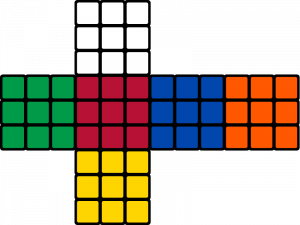First, similar to a programming language, let’s establish the notation we use:

Each of the 6 cube faces will be given the first letter of their name:

1. U – Up
2. D – Down
3. L – left
4. R – right
5. F – front
6. B – back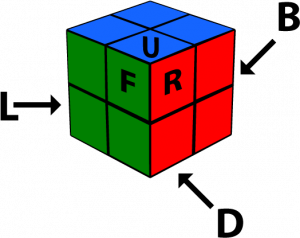Also, we need to have in mind that each face can be turned in 3 directions.

Here’s an example using L on how this is represented:

1. L – clockwise quarter-turn of the upper face
2. L’ – counter-clockwise quarter-turn of the upper face
3. L2 – half turn in either direction of the upper face

Now grab a Rubik’s Cube and follow this algorithm with your students to solve it:

Step 1 – The Plus:

1. Turn some edges until you have a white plus on the upper face
2. Do the following turns F, R’, D’, R, F2, R’, U, R, U’, R’, R2, L2, U2, R2, L2
3. The plus should now be solved.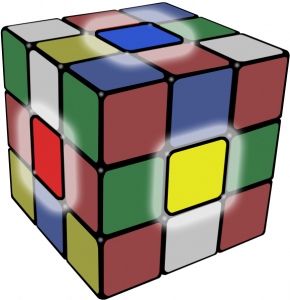Step 2 – The Corners

1. While the edges on the white face are complete, the corners are still not done.
2. Based on where the white-orange-green corner is in the puzzle, use one of the following turning algorithms:
3. Bottom: R’, D’, R, D – repeat until the corner is in correct position
4. Top: R’, D’, R, D – once the corner is to the bottom, follow the above instructions

Step 3: Middle Layer Edges

1. Turn the cube so the white is on the bottom
2. Find an edge that is on top face without yellow on it
3. Do a U-turn so that the color on the front face of the edge matches the center
4. Based on direction of the edge can go, use one of the following turning algorithms:
5. Left: U’, L’, U, L, U, F, U’, F’
6. Right: U, R, U’, R’, U’, F’, U, F

Step 4: Yellow Plus

1. Do the following turns until a yellow cross appears with the yellow center: F, R, U, R’, U’, F’
2. If an “L” shape is seen where the two yellow pieces are adjacent to each other, do the following turns: F, U, R, U’, R’, F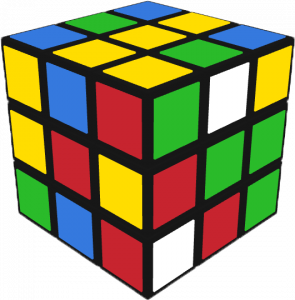1. If a horizontal “Line” shape is seen, do the following turns: F, R, U, R’, U’, F’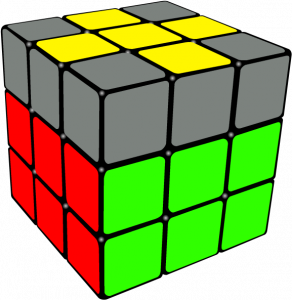Step 5: Sune and Anti-Sune1  algorithms

1 Sune and Anti-Sune – Sune is a term used in Rubik’s Cube when the edges are oriented and three corners are twisted counterclockwise – also being the name of the algorithm which solves it.

1. Find the face with the yellow center.
2. Based on the below occurrences, apply one of the following series of turns:
1. For only one oriented corner: R, U, R’, U, R, U2, R’ – repeat until correct position
2. For one oriented corner and one right-facing corner: U2, R, U2, R’, U’, R, U’, R’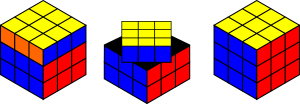Step 6: Solving the cube

1. Look for sets of “split matches” – two squares in the of the same color in the same row, separated by a sticker of a different color
2. Based on how many there are, use one of the following turning algorithms:
3. If you see a set of split matches on each side: R, U’, R, U, R, U, R, U’, R’, U’, R2
4. If not, use: R’, F, R’, B2, R, F’, R’, B2, R2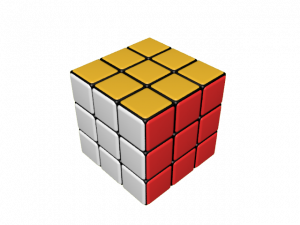The Rubik’s Cube should now be solved!

At the end, why not offer an extra incentive to your students and check with them the Chrome Cube Lab that offers various puzzles and interactive objects based on Rubik’s Cube:

www.chrome.com/cubelab#demo

As you know, algorithm thinking is essential in all technical fields, from machine learning to artificial intelligence.

Teaching your students basic algorithm concepts will allow them to break down problems and conceptualize solutions in terms of concrete steps.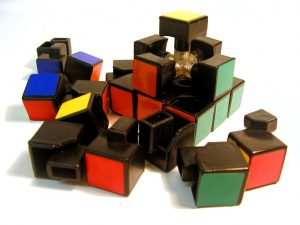Have fun with the Rubik’s Cube and its many algorithms, and don’t forget to share the results!